## RS Aggarwal Class 6 Solutions Chapter 5 Fractions Ex 5D

These Solutions are part of RS Aggarwal Solutions Class 6. Here we have given RS Aggarwal Solutions Class 6 Chapter 5 Fractions Ex 5D.

Other Exercises

Question 1.
Solution:
(i) Like fraction : Fractions having the same denominators are called like fractions. For examples:
$$\frac { 2 }{ 11 } ,\frac { 3 }{ 11 } ,\frac { 4 }{ 11 } ,\frac { 5 }{ 11 } ,\frac { 8 }{ 11 }$$
(ii) Unlike fraction : Fraction having the different denominators, are called unlike fractions. For examples:
$$\frac { 1 }{ 3 } ,\frac { 4 }{ 7 } ,\frac { 5 }{ 9 } ,\frac { 3 }{ 8 } ,\frac { 7 }{ 11 }$$

Question 2.
Solution:
We know that like fractions have same denominator
Now $$\frac { 3 }{ 5 } ,\frac { 7 }{ 10 } ,\frac { 8 }{ 15 } ,\frac { 11 }{ 30 }$$
LCM of 5, 10, 15 and 30 = 30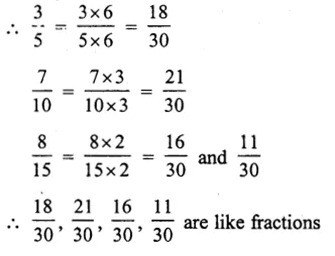Question 3.
Solution:
We know that like fraction have same denominators
$$\frac { 1 }{ 4 } ,\frac { 5 }{ 8 } ,\frac { 7 }{ 12 } ,\frac { 13 }{ 24 }$$
LCM of 4, 8, 12, 24 = 24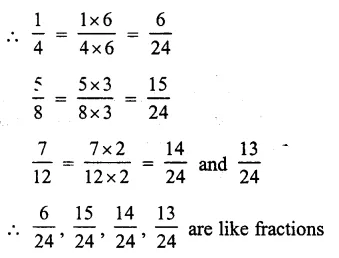Question 4.
Solution: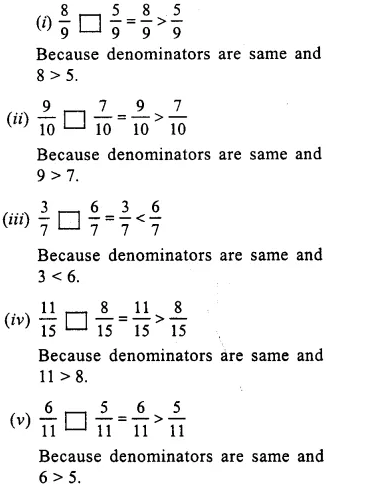Question 5.
Solution: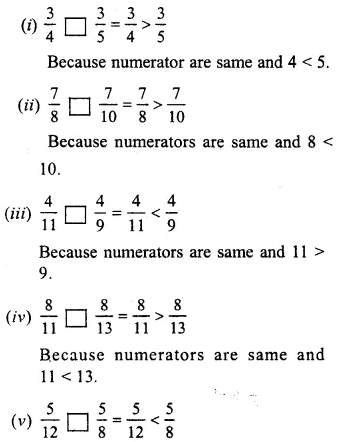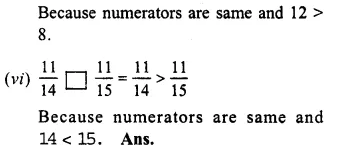Compare the fractions given below :

Question 6.
Solution:
$$\frac { 4 }{ 5 } and\frac { 5 }{ 7 }$$
LCM of 5 and 7 = 35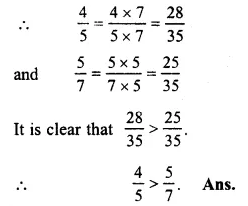Question 7.
Solution:
$$\frac { 3 }{ 8 } and\frac { 5 }{ 6 }$$
LCM of 8 and 6 = 24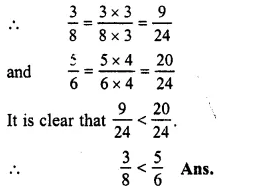Question 8.
Solution:
$$\frac { 7 }{ 11 } and\frac { 6 }{ 7 }$$
LCM of 11 and 7 = 77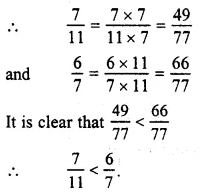Question 9.
Solution:
$$\frac { 5 }{ 6 } and\frac { 9 }{ 11 }$$
LCM of 6 and 11 = 66Question 10.
Solution:
$$\frac { 2 }{ 3 } and\frac { 4 }{ 9 }$$
LCM of 3 and 9 = 9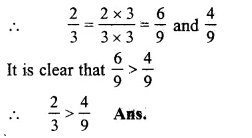Question 11.
Solution:
$$\frac { 6 }{ 13 } and\frac { 3 }{ 4 }$$
LCM of 13 and 4 = 52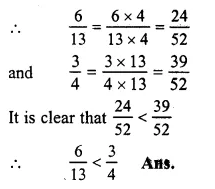Question 12.
Solution:
$$\frac { 3 }{ 4 } and\frac { 5 }{ 6 }$$
LCM of 4 and 6 = 12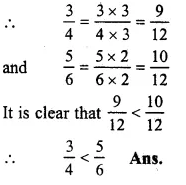Question 13.
Solution:
$$\frac { 5 }{ 8 } and\frac { 7 }{ 12 }$$
LCM of 8 and 12 = 24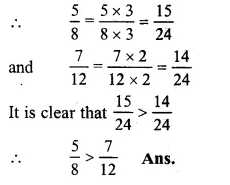Question 14.
Solution:
$$\frac { 4 }{ 9 } and\frac { 5 }{ 6 }$$
LCM of 9 and 6 = 18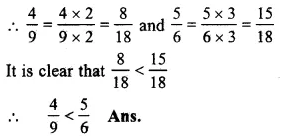Question 15.
Solution:
$$\frac { 4 }{ 5 } and\frac { 7 }{ 10 }$$
LCM of 5 and 10 = 10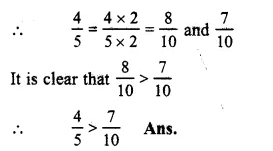Question 16.
Solution:
$$\frac { 7 }{ 8 } and\frac { 9 }{ 10 }$$
LCM of 8 and 10 = 40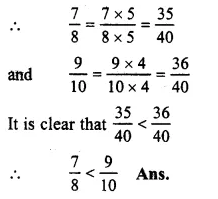Question 17.
Solution:
$$\frac { 11 }{ 12 } and\frac { 13 }{ 15 }$$
LCM of 12 and 15 = 60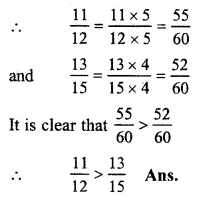Question 18.
Solution:
$$\frac { 1 }{ 2 } ,\frac { 3 }{ 4 } ,\frac { 5 }{ 6 } and\frac { 7 }{ 8 }$$
LCM of 2, 4, 6 and 8 = 24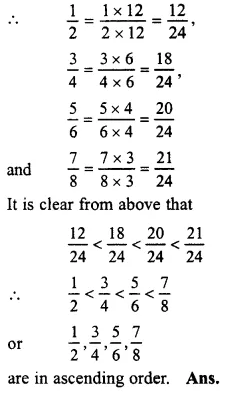Question 19.
Solution:
$$\frac { 2 }{ 3 } ,\frac { 5 }{ 6 } ,\frac { 7 }{ 9 } and\frac { 11 }{ 18 }$$
LCM of 3, 6, 9 and 18 = 18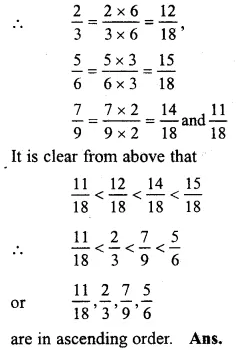Question 20.
Solution:
$$\frac { 2 }{ 5 } ,\frac { 7 }{ 10 } ,\frac { 11 }{ 15 } and\frac { 17 }{ 30 }$$
LCM of 5, 10, 15 and 30 = 30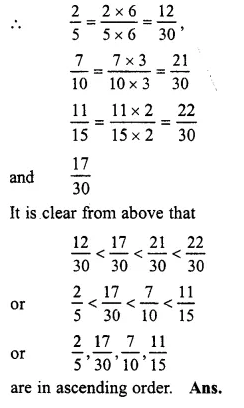Question 21.
Solution:
$$\frac { 3 }{ 4 } ,\frac { 7 }{ 8 } ,\frac { 11 }{ 16 } and\frac { 23 }{ 32 }$$
LCM of 4, 8, 16 and 32 = 32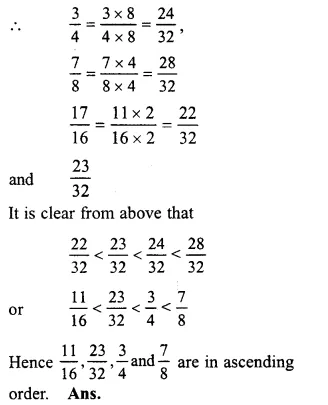Arrange the following fractions in the descending order :

Question 22.
Solution:
$$\frac { 3 }{ 4 } ,\frac { 5 }{ 8 } ,\frac { 11 }{ 12 } and\frac { 17 }{ 24 }$$
LCM of 4, 8, 12 and 24 = 24Question 23.
Solution:
$$\frac { 7 }{ 9 } ,\frac { 5 }{ 12 } ,\frac { 11 }{ 18 } and\frac { 17 }{ 36 }$$
LCM of 9, 12, 18 and 36 = 36Question 24.
Solution:
$$\frac { 2 }{ 3 } ,\frac { 3 }{ 5 } ,\frac { 7 }{ 10 } and\frac { 8 }{ 15 }$$
LCM of 3, 5, 10 and 15 = 30Question 25.
Solution:
$$\frac { 5 }{ 7 } ,\frac { 9 }{ 14 } ,\frac { 17 }{ 21 } and\frac { 31 }{ 42 }$$
LCM of 7, 14, 21 and 42 = 42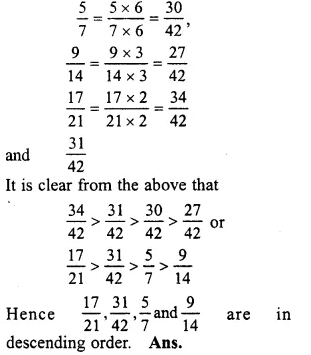Question 26.
Solution:
∴ the numerators are equal
∴ The fraction having small denominator is greater than the fraction having large denominator
∴ In descending order, we can write
$$\frac { 1 }{ 12 } ,\frac { 1 }{ 23 } ,\frac { 1 }{ 7 } ,\frac { 1 }{ 9 } ,\frac { 1 }{ 17 } ,\frac { 1 }{ 50 }$$

Question 27.
Solution:
Here, the numerators of all fractions are equal
∴ The fraction having small denominator is greater than the fraction having large denominator
Now in descending order is
$$\frac { 3 }{ 4 } ,\frac { 3 }{ 5 } ,\frac { 3 }{ 7 } ,\frac { 3 }{ 11 } ,\frac { 3 }{ 13 } ,\frac { 3 }{ 17 }$$

Question 28.
Solution:
Lalita read 30 pages of a book containing 100 pages
She read $$\\ \frac { 30 }{ 100 }$$ = $$\\ \frac { 3 }{ 10 }$$ part of the book and Sarita read $$\\ \frac { 2 }{ 5 }$$ of the book
Now in $$\\ \frac { 3 }{ 10 }$$ and $$\\ \frac { 2 }{ 5 }$$, LCM of 10, 5 = 10
$$\\ \frac { 3 }{ 10 }$$ = $$\\ \frac { 3 }{ 10 }$$
$$\\ \frac { 2 }{ 5 }$$ = $$\\ \frac { 2\times 2 }{ 5\times 2 }$$ = $$\\ \frac { 4 }{ 10 }$$
as $$\\ \frac { 4 }{ 10 }$$ or $$\frac { 2 }{ 5 } >\frac { 3 }{ 10 }$$

Question 29.
Solution:
Rafiq exercised for $$\\ \frac { 2 }{ 3 }$$ hour and Rohit exercised for $$\\ \frac { 3 }{ 4 }$$ hour
In $$\\ \frac { 2 }{ 3 }$$ and $$\\ \frac { 3 }{ 4 }$$, LCM of 3 and 4 = 12
$$\\ \frac { 2 }{ 3 }$$ = $$\\ \frac { 2\times 4 }{ 3\times 4 }$$ = $$\\ \frac { 8 }{ 12 }$$
$$\\ \frac { 3 }{ 4 }$$ = $$\\ \frac { 3\times 3 }{ 4\times 3 }$$ = $$\\ \frac { 9 }{ 12 }$$
$$\frac { 9 }{ 12 } >\frac { 8 }{ 12 }$$
=> $$\frac { 3 }{ 4 } >\frac { 2 }{ 3 }$$
∴ Rohit exercised more time

Question 30.
Solution:
In VI A, 20 student passed out of 25 or $$\\ \frac { 20 }{ 25 }$$ or $$\\ \frac { 4 }{ 5 }$$ students passed
But in VI B, 24 out of 30 passed 24 or $$\\ \frac { 24 }{ 30 }$$ or $$\\ \frac { 4 }{ 5 }$$ students passed
Now $$\\ \frac { 4 }{ 5 }$$ = $$\\ \frac { 4 }{ 5 }$$
∴ Both sections gave same result

Hope given RS Aggarwal Solutions Class 6 Chapter 5 Fractions Ex 5D are helpful to complete your math homework.

If you have any doubts, please comment below. Learn Insta try to provide online math tutoring for you.